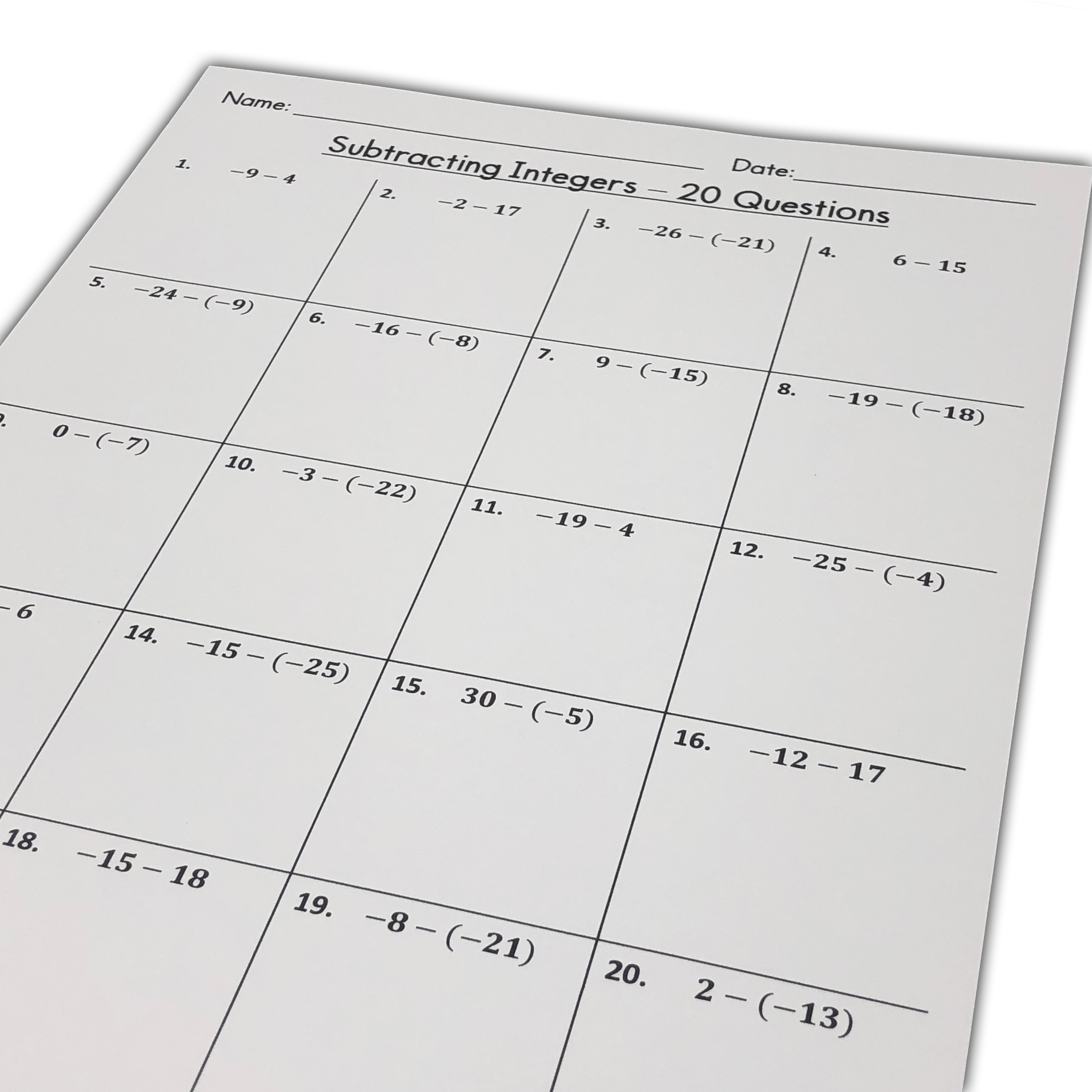# FREE Beginners Subtracting Integers Worksheets (Integers from -30 to 30)

### Are you looking for simple subtracting integers worksheets for your 6th, 7th, or 8th grade math class?

Why not use these free worksheets?

I created three no-frills subtracting integers worksheets for you to use, with answer keys included!

### These are beginner level worksheets.

Each expression contains two integers between -30 and 30.

These basic subtracting integers problems are perfect for your students who are just beginning to learn integer operations.

### Want to try some problems that are a little easier?

Try my subtracting integers worksheets with integers between -10 and 10.